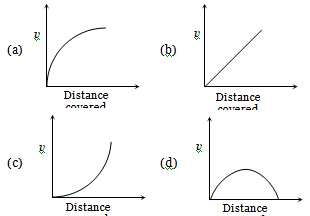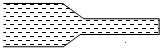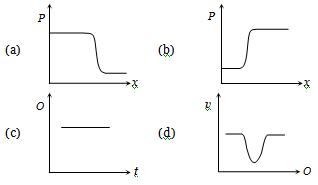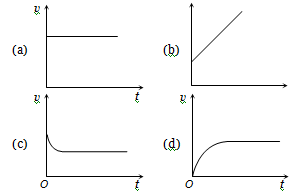A block of ice floats on a liquid of density 1.2 $g/c{m}^{3}$ in a beaker. Then level of liquid when ice completely melts-

(a) Remains same                         (b) Rises
(c) Lowers                                    (d) (a), (b) or (c)

Concept Questions :-

Archimedes principle
High Yielding Test Series + Question Bank - NEET 2020

Difficulty Level:

A vessel of area of cross-section A has liquid to a height H. There is a hole at the bottom of vessel having area of cross-section a. The time taken to decrease the level from ${\mathrm{H}}_{1}$ to ${\mathrm{H}}_{2}$ will be

(a)$\frac{\mathrm{A}}{\mathrm{a}}\sqrt{\frac{2}{\mathrm{g}}}\left[\sqrt{{\mathrm{H}}_{1}}-\sqrt{{\mathrm{H}}_{2}}\right]$              (b) $\sqrt{2\mathrm{gh}}$

(c) $\sqrt{2\mathrm{gh}\left({\mathrm{H}}_{1}-{\mathrm{H}}_{2}\right)}$                      (d) $\frac{\mathrm{A}}{\mathrm{a}}\sqrt{\frac{g}{2}}\left[\sqrt{{\mathrm{H}}_{1}}-\sqrt{{\mathrm{H}}_{2}}\right]$

Concept Questions :-

Bernoulli theorem
High Yielding Test Series + Question Bank - NEET 2020

Difficulty Level:

A lead shot of 1mm diameter falls through a long column of glycerine. The variation of its velocity v with distance covered is represented byConcept Questions :-

Viscosity
High Yielding Test Series + Question Bank - NEET 2020

Difficulty Level:

Water flows through a frictionless duct with a cross-section varying as shown in fig. Pressure p at points along the axis is represented byConcept Questions :-

Bernoulli theorem
High Yielding Test Series + Question Bank - NEET 2020

Difficulty Level:

From amongst the following curves, which one shows the variation of the velocity v with time t for a small sized spherical body falling vertically in a long column of a viscous liquid -Concept Questions :-

Viscosity
High Yielding Test Series + Question Bank - NEET 2020

Difficulty Level:

A liquid does not wet the sides of a solid if the angle of contact is

(a) Zero                                     (b) Obtuse (More than 90°)
(c) Acute (Less than 90°)            (d) 90°

Concept Questions :-

Surface tension
High Yielding Test Series + Question Bank - NEET 2020

Difficulty Level:

The meniscus of mercury in the capillary tube is

(a) Convex            (b) Concave
(c) Plane               (d) Uncertain

Concept Questions :-

Capillary rise
High Yielding Test Series + Question Bank - NEET 2020

Difficulty Level:

At which of the following temperatures, the value of surface tension of water is minimum ?

(a) $4°\mathrm{C}$                                       (b) $25°\mathrm{C}$
(c) $50°\mathrm{C}$                                     (d) $75°\mathrm{C}$

Concept Questions :-

Surface tension
High Yielding Test Series + Question Bank - NEET 2020

Difficulty Level:

If a glass rod is dipped in mercury and withdrawn out, the mercury does not wet the rod because

(a) Angle of contact is acute

(b) Cohesion force is more

(d) The density of mercury is more

Concept Questions :-

Surface tension
High Yielding Test Series + Question Bank - NEET 2020

Difficulty Level:

Mercury does not wet glass, wood or iron because

(a) Cohesive force is less than the adhesive force

(b) Cohesive force is greater than the adhesive force

(c) The angle of contact is less than 90o

(d) Cohesive force is equal to the adhesive force

Concept Questions :-

Surface tension